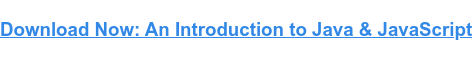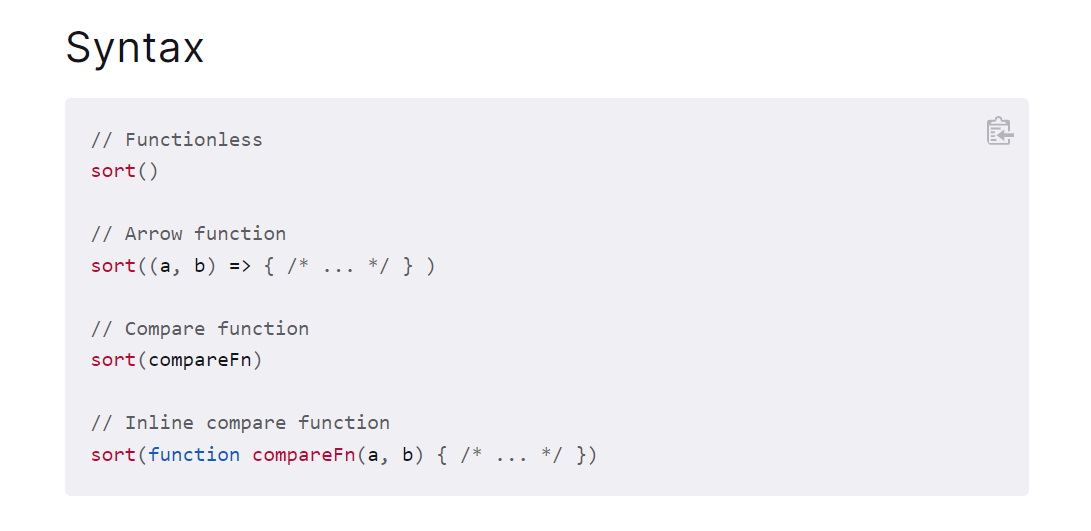# An Introduction to the JavaScript Array Sort MethodUpdated:

Published:

When programming, developers often need a way to sort and organize information. JavaScript provides a variety of ways to do so.This post will discuss a method used to sort through array items. The sort() method can be used on arrays with different kinds of data. Below, you’ll learn about the array sort method and how it works, as well as look at some examples.

Without any further delay, let's jump right into it.

##hbspt.cta._relativeUrls=true;hbspt.cta.load(53, 'b788eafd-18fb-4007-bfc9-f22703266f85', {"useNewLoader":"true","region":"na1"}); Javascript Sort

Developers often work with large amounts of data represented by different types of objects. No matter the form or size of the data collection, it will need to be sorted or organized.

There are different ways to accomplish this depending on the data management case. For example, you may need to write your own custom algorithm to sort or organize the data. In other instances, JavaScript provides the tools you need.

## Sort Method JavaScript

The JavaScript sort method follows most of the same syntax rules of any other method; it is chained to the object you want it to run on. The sort method also accepts an optional parameter for comparing array items.## How the JavaScript Array Sort Method Works

In its simplest form, the sort method will look through the objects and sort them based on which item comes first. This behavior is true for both integers and strings; in either case, it will look at the items and discern what order to organize them.Noteworthy Caveat: It is worth noting that if you have array items that are numbers (integers represented as strings, i.e., “23”), the sort method will only look at the first number in the string. This may present unpredictable results, especially when using the optional compare function parameter.

## JavaScript Sort Array of Objects

You’ve learned a lot about the sort method, but nothing can quite compare to seeing it used in practice.

Let's look at a few examples of using the sort method, starting with how to use it with strings.

### Array of Strings

Take this code example below:

` `const fruits = ["Banana", "Orange", "Apple", "Mango"];fruits.sort();``

The result of the above code would return — [Apple, Banana, Mango, Orange] — which is the alphabetical order of the items. This is a straightforward use of the sort method, though it is interesting to note that this works with const variable types. This is because const variables are constant and cannot be altered; however, changing the order of the items inside does not change the item, so this approach still works.

### Array of Numbers

This approach also works for strings containing numerical characters and integers as well. So let’s look at the example below.

The above code would return the strings in the proper numerical order; however, the result would be different if you replaced one of the items with the number 100. Changing the numbers to 100 would result in that number being placed first since the first number is one and the rest are zeros. The behavior would still be the same with a mixture of string numbers and integers.

### The Compare Function

The last thing to showcase here is how to use the sort method using its compare function parameter.

` `myVar.sort(function(a, b){return a-b});``

The sort method’s compare function parameter has a simple syntax; it accepts a function that takes two arguments and returns a positive, zero, or negative value. Based on the return value, it will assign a position for each item in the array. If the return value is a negative number, then the sort method knows the first number compared is lower than the second number.

Likewise, if the return value is positive, then the first number is higher, and a return value of zero implies the two values are the same.

Let’s look at an example of using the function to create a numerically organized array of numbers.

` `const points = [40, 100, 1, 5, 25, 10];points.sort(function(a, b){return a-b});``

The above code would return the reorganized array with the following sequence [1,5,10,25,40,100]; the array gets restructured in ascending order, lowest to highest.

## Using the Sort Method in JavaScript

You’ve learned a lot about how the JavaScript array sort method works; with this information, you are now ready to start using the sort method on your arrays. There are many uses for the sort method, such as organizing scores in a game to decide who won. Be sure to practice using it to learn its limitations and application.Topics: Javascript

## Related Articles

We're committed to your privacy. HubSpot uses the information you provide to us to contact you about our relevant content, products, and services. You may unsubscribe from these communications at any time. For more information, check out our Privacy Policy.